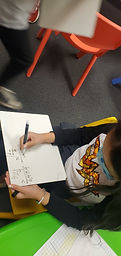Mr. Jake

Target 1​

Lesson Type:

Review

Number Operation

:

Computation

Multiply within 1,000.

1:

Understand there are many ways to multiply multi-digit numbers.

2:

Explain how different methods produce the same product (how each method is doing the same thing).

3:

Understand the role of place-value in multiplication strategies.

4:

Use the standard algorithm for solving multi-digit multiplication equations.

4th

Vocabulary:

Multiplication, Product, Factor

Activities:

Practiced solving multiplication problems with multiple methods

Created multiplication problems using dice and determined the product.Home Exploration

Guiding Questions:Absent Students:

Target 2

:

1:

Define reflectional symmetry.

5th

Vocabulary:

Reflection, Axis, Coordinates

Activities:

Created shapes on the coordinate plane and reflected the shapes across the X and Y axis.Home Exploration

Guiding Questions:Target 3

:

Vocabulary:

Activities:Home Exploration